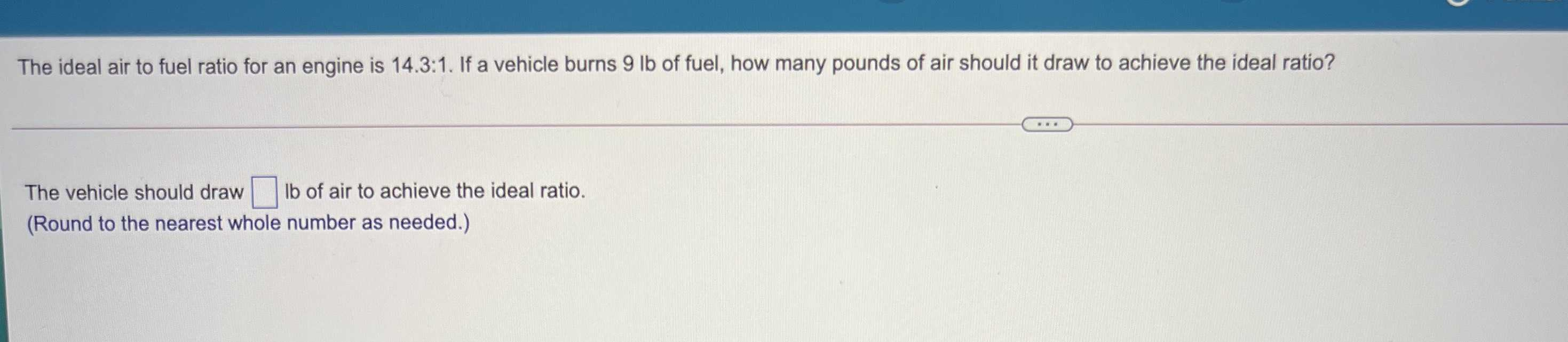### Still have math questions?

Algebra
QuestionThe ideal air to fuel ratio for an engine is $$14.3 : 1$$ . If a vehicle burns $$9 lb$$ of fuel, how many pounds of air should it draw to achieve the ideal ratio?

The vehicle should draw $$\square Ib$$ of air to achieve the ideal ratio.

(Round to the nearest whole number as needed.)